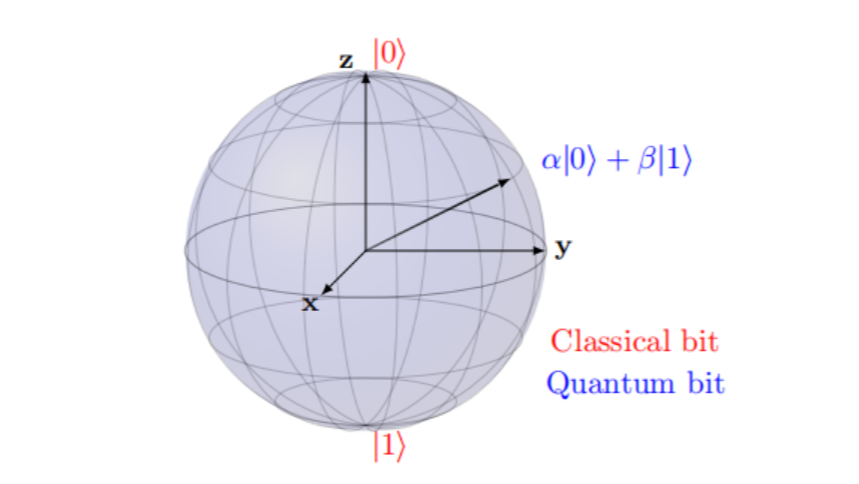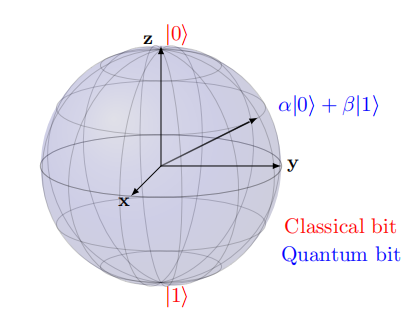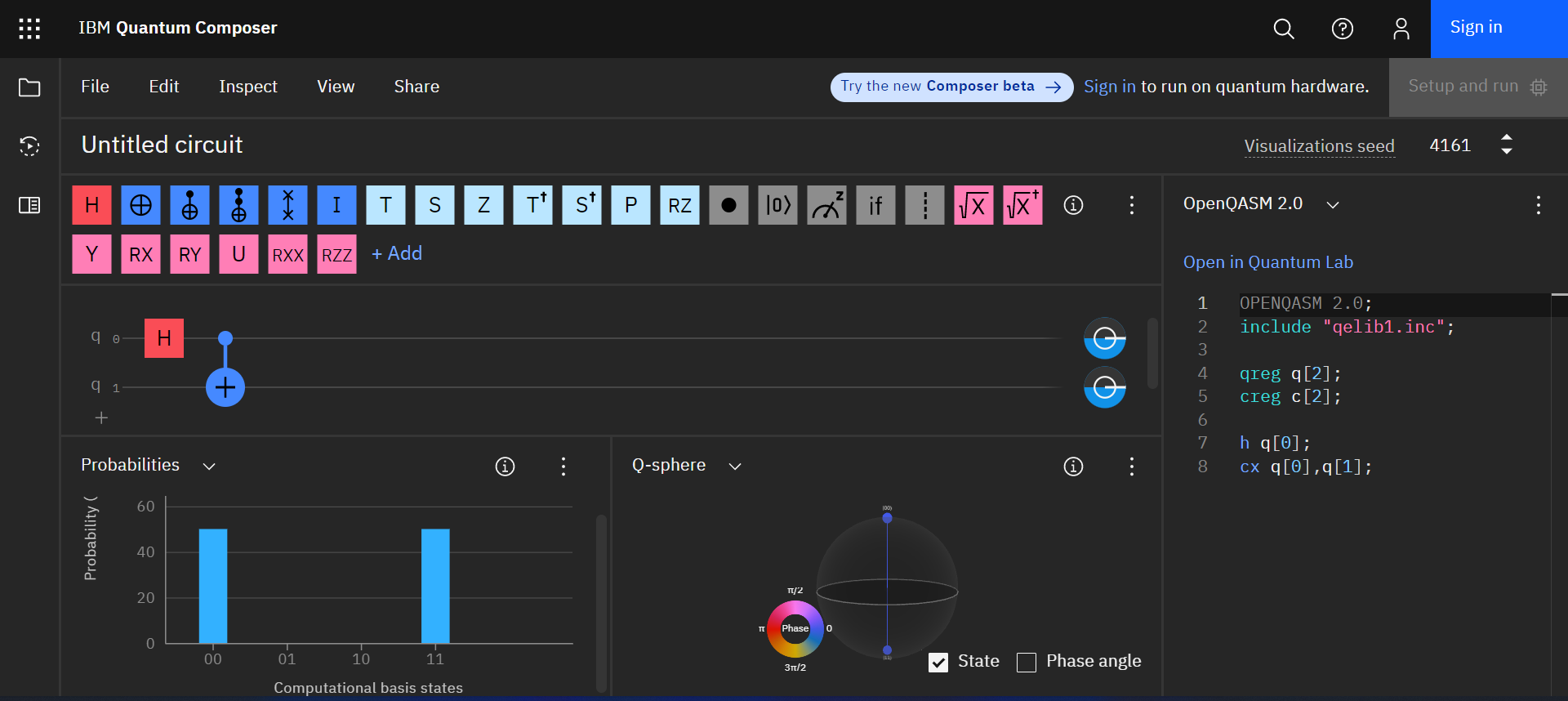# 量子计算基础——矩阵语言量子计算是一门当下非常火热的技术，抛开个别企业对量子计算的过分吹嘘不谈，其本身是一门非常有意义的跨学科研究领域。本文仅从非物理科班专业的角度——用矩阵的语言去描述量子计算的基础单元和基础操作，包含量子态的含义、单比特量子门操作以及两比特量子门操作的矩阵形式。并且附带一定的物理图像，这一点其实非常重要，如果不断的推导公式，最终有可能迷失了其物理图像，这就脱离了我们做研究的初衷。

# 技术背景# 量子比特与量子操作

$\left|\psi\right>=\left[ \begin{matrix} p_0\\ p_1 \end{matrix} \right]$

$\left|0\right>=\left[ \begin{matrix} 1\\0 \end{matrix} \right],\left|1\right>=\left[ \begin{matrix} 0\\1 \end{matrix} \right]$

$\left|\psi\right>=p_0\left|0\right>+p_1\left|1\right>$

$p_0^2+p_1^2=1$

$\left|\psi\right>=p_{00}\left|00\right>+p_{01}\left|01\right>+p_{10}\left|10\right>+p_{11}\left|11\right>\\ \left|\psi\right>=p_{000}\left|000\right>+p_{001}\left|001\right>+p_{010}\left|010\right>+p_{011}\left|011\right>+p_{100}\left|100\right>+p_{101}\left|101\right>+p_{110}\left|110\right>+p_{111}\left|111\right>$

$\left|\psi\right>=p_0\left|0\right>+p_1\left|1\right>=(p_{x0}+ip_{y0})\left|0\right>+(p_{x1}+ip_{y1})\left|1\right>$

$\sigma^I=\left( \begin{array}{1} 1 & 0\\ 0 & 1 \end{array} \right), \sigma^X=\left( \begin{array}{1} 0 & 1\\ 1 & 0 \end{array} \right), \sigma^Y=\left( \begin{array}{1} 0 & -i\\ i & 0 \end{array} \right), \sigma^Z=\left( \begin{array}{1} 1 & 0\\ 0 & -1 \end{array} \right)$

$e^{A}=\sum^{\infty}_{k=0}\frac{1}{k!}A^{k}$

$R_M(\theta)=e^{-i\theta\sigma^M}=cos\left(\frac{\theta}{2}\right)\sigma^I-isin\left(\frac{\theta}{2}\right)M,M\in\left(\sigma^X,\sigma^Y,\sigma^Z\right)$

$H=\frac{\sqrt{2}}{2}(\sigma^X+\sigma^Z)$

$P(\theta)=\left( \begin{matrix} 1&0\\ 0&e^{i\theta} \end{matrix} \right)$

\begin{align*} \left|\psi\right>&=e^{i\phi_1}cos\frac{\theta}{2}\left| 0\right>+e^{i\phi_2}sin\frac{\theta}{2}\left| 1\right>\\ &=e^{i\phi_1}(cos\frac{\theta}{2}\left| 0\right>+e^{i(\phi_2-\phi_1)}sin\frac{\theta}{2}\left| 1\right>)\\ &=cos\frac{\theta}{2}\left| 0\right>+e^{i(\phi_2-\phi_1)}sin\frac{\theta}{2}\left| 1\right>\\ &=\bold{\psi}(cos\phi sin\theta,sin\phi sin\theta, cos\theta) \end{align*}

$CX=\left|0\right>\left<0\right|\cdot I+\left|1\right>\left<1\right|\cdot \sigma^X=\left( \begin{array}{1} 1 & 0 & 0 & 0\\ 0 & 1 & 0 & 0\\ 0 & 0 & 0 & 1\\ 0 & 0 & 1 & 0 \end{array} \right)$$H\left|0\right>=\frac{\sqrt{2}}{2}\left( \begin{matrix} 1&1\\ 1&-1 \end{matrix} \right)\left( \begin{matrix} 1\\ 0 \end{matrix} \right)=\left( \begin{matrix} \frac{\sqrt{2}}{2}\\ \frac{\sqrt{2}}{2} \end{matrix} \right)$

$\left|\psi_H\right>=\left( \begin{matrix} \frac{\sqrt{2}}{2}\\ \frac{\sqrt{2}}{2} \end{matrix} \right)\otimes\left( \begin{matrix} 1\\ 0 \end{matrix} \right)= \left( \begin{matrix} \frac{\sqrt{2}}{2}\\ 0\\ \frac{\sqrt{2}}{2}\\ 0 \end{matrix} \right)$

$\left|\psi_{final}\right>=CX\left|\psi_H\right>=\left( \begin{array}{1} 1 & 0 & 0 & 0\\ 0 & 1 & 0 & 0\\ 0 & 0 & 0 & 1\\ 0 & 0 & 1 & 0 \end{array} \right)\left( \begin{matrix} \frac{\sqrt{2}}{2}\\ 0\\ \frac{\sqrt{2}}{2}\\ 0 \end{matrix} \right)= \left( \begin{matrix} \frac{\sqrt{2}}{2}\\ 0\\ 0\\ \frac{\sqrt{2}}{2} \end{matrix} \right)$

$Swap=\left( \begin{array}{1} 1 & 0 & 0 & 0\\ 0 & 0 & 1 & 0\\ 0 & 1 & 0 & 0\\ 0 & 0 & 0 & 1 \end{array} \right)$

# 参考文献

1. Quantum Computational Chemistry. Sam McArdle, Suguru Endo and other co-authors.
2. The Basics of Quantum Computing for Chemists. Daniel Claudino.
3. https://quantum-computing.ibm.com/composer/
4. https://www.quantum-inspire.com/kbase/rz-gate/

1 total views,  1 views today